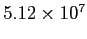Performance of the Hybrid Parallel CABARET code

In this section we shall discuss the performance of the hybrid parallel CABARET. We shall revisit thecell backward facing step, again with boundary conditions for laminar flow, Reynolds number=5000 and Mach number=0.1. For each test run we can vary the number MPI processes (n), the number MPI processes per node (N) and the number of OpenMP threads per MPI process (d). There is always one MPI process per hex core die or per socket.

Figureshows the speedup for 50, 250, 500 and 1000 MPI processes for 276 time steps. A comparison can be made between the two runs, which are shown for:

1. 4 MPI processes per node with 6 OpenMP threads per MPI process.
2. 2 MPI processes per node with 12 OpenMP threads per MPI process.

The two runs were carried out on HECToR Phase 2b with the Seastar2+ interconnect. Case 1. performs slightly better than Case 2. This is a demonstrative case and for a variation in problem size, the situation may be slightly different. The ratio between computation and communication, is an important factor in CABARET performance since the main computational loops are non vectorised. So for any particular problem size, it would be recommended to test which setup gives best performance for the number MPI processes per node and the number of OpenMP threads per MPI process for a given number MPI processes (partitions). It's worthwhile to note that performing the same simulation with the Gemini interconnect does give a 5-10% speedup, which is expected since this computation is using a one dimensional data decomposition and is not heavily dependent upon the performance of communication.Phil Ridley 2011-02-01